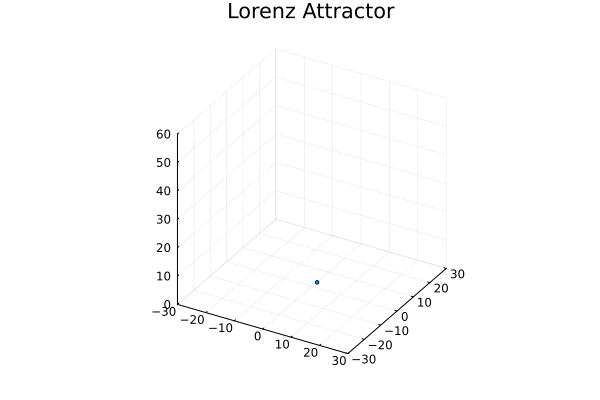# Lorenz Attractorusing Plots
gr()

# define the Lorenz attractor
Base.@kwdef mutable struct Lorenz
dt::Float64 = 0.02
σ::Float64 = 10
ρ::Float64 = 28
β::Float64 = 8 / 3
x::Float64 = 1
y::Float64 = 1
z::Float64 = 1
end

function step!(l::Lorenz)
dx = l.σ * (l.y - l.x)
dy = l.x * (l.ρ - l.z) - l.y
dz = l.x * l.y - l.β * l.z
l.x += l.dt * dx
l.y += l.dt * dy
l.z += l.dt * dz
end

attractor = Lorenz()

# initialize a 3D plot with 1 empty series
plt = plot3d(
1,
xlim = (-30, 30),
ylim = (-30, 30),
zlim = (0, 60),
title = "Lorenz Attractor",
legend = false,
marker = 2,
)

# build an animated gif by pushing new points to the plot, saving every 10th frame
# equivalently, you can use @gif to replace @animate and thus no need to explicitly call gif(anim).
anim = @animate for i = 1:1_500
step!(attractor)
push!(plt, attractor.x, attractor.y, attractor.z)
end every 10
gif(anim)